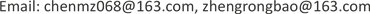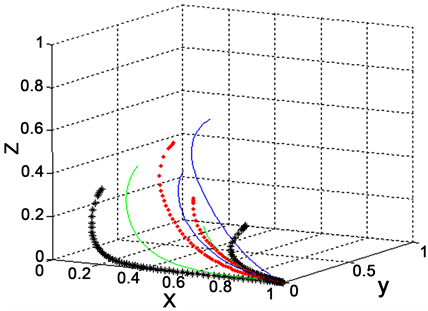﻿ 土地合作社制度下的收益分配主体间演化博弈分析 The Evolutionary Game Analysis of Enterprise’s Income Distribution in Land Joint-Stock Cooperative System Reform

Vol. 08  No. 01 ( 2019 ), Article ID: 28748 , 8 pages
10.12677/ASS.2019.81025

The Evolutionary Game Analysis of Enterprise’s Income Distribution in Land Joint-Stock Cooperative System Reform

Meizhao Chen1, Rongbao Zheng2, Yaqi Zhang2

1School of Social and Public Administration, Guangdong University of Foreign Studies, Guangzhou Guangdong

2College of Management , Guangdong University of Technology, Guangzhou GuangdongReceived: Jan. 10th, 2019; accepted: Jan. 24th, 2019; published: Jan. 31st, 2019ABSTRACT

The land joint-stock cooperative system reform can change the existing business model of rural collective land, and rise peasants’ income, however, the resulting problem of inequalities of land revenue distribution is increasingly more serious. Taking Longdong village in Tianhe district of Guangzhou city as an example, this paper builds a pay off matrix model among government, economic association and villagers based on replicated dynamic model, and applies the Jacobi iteration method to analyze the stability of the equilibrium point. Model results show that the system of equations is in a stable state when equilibrium pay off matrix equation of the three groups reaches [1,0,0]. Thus, the government, economic association and villagers make decision to the strategy combination {regulation, unsustainable management, silence}. We find that although government takes regulation, the economic association lacks of standard operation. Moreover, the villagers are often short of protection of rights when they face damages on rights and interests.

Keywords:Land, Joint Stock Cooperation, Income Distribution, Stakeholders, Evolutionary Game Theory

1广东外语外贸大学，社会与公共管理学院，广东 广州

2广东工业大学，管理学院，广东 广州1. 引言

2. 研究区土地股份合作制概况

3. 收益分配主体演化博弈模型

3.1. 基本假设及参数假设Table 1. Return matrix of the government, economic association and villager

3.2. 演化博弈模型的建立

${V}_{G1}=\left({S}_{1}+T-{C}_{1}-{C}_{2}\right)y+\left({S}_{1}z+{S}_{2}-{C}_{1}-{C}_{2}\right)\left(1-y\right)$ (1)

${V}_{G2}=-{C}_{2}zy-{S}_{1}z$ (2)

$\stackrel{¯}{{V}_{G}}={V}_{G1}x+{V}_{G2}\left(1-x\right)$ (3)

$U\left(x\right)=\frac{\text{d}x}{\text{d}t}=\left[\left(T-{S}_{2}+{C}_{2}\right)y+{S}_{2}-{C}_{1}-{C}_{2}\right]x\left(1-x\right)$ (4)

$U\left(y\right)=\frac{\text{d}y}{\text{d}t}=\left[\left({S}_{2}-T\right)x+\left(R-{D}_{1}\right)z+{I}_{1}-{I}_{2}\right]y\left(1-y\right)$ (5)

$U\left(z\right)=\frac{\text{d}z}{\text{d}t}=\left[{C}_{2}x+\left({D}_{1}-{C}_{3}+{C}_{2}\right)y-{C}_{5}-{C}_{2}+{C}_{4}\right]z\left(1-z\right)$ (6)

$\frac{\text{d}x}{\text{d}t}$$\frac{\text{d}y}{\text{d}t}$$\frac{\text{d}z}{\text{d}t}$ 均为零，可通过Mathematics 9.0计算得出复制者动态的均衡点。演化稳定策略要求，倘若，则是演化稳定策略，即ESS。 ${E}_{1}=\left[0,0,0\right]$${E}_{2}=\left[1,0,0\right]$${E}_{3}=\left[0,1,0\right]$${E}_{4}=\left[1,1,0\right]$${E}_{5}=\left[0,0,1\right]$${E}_{6}=\left[1,0,1\right]$${E}_{7}=\left[0,1,1\right]$${E}_{8}=\left[1,1,1\right]$

${E}_{9}=\left[0,-\left({I}_{2}-{I}_{1}\right){\left(T-{S}_{2}\right)}^{-1},0\right]$${E}_{10}=\left[1,-\left({I}_{2}-{I}_{1}\right){\left(T-{S}_{2}\right)}^{-1},0\right]$${E}_{11}=\left[0,-\left(D{}_{1}-R-{I}_{1}+{I}_{2}\right){\left(T-{S}_{2}\right)}^{-1},1\right]$

${E}_{12}=\left[1,-\left(D{}_{1}-R-{I}_{1}+{I}_{2}\right){\left(T-{S}_{2}\right)}^{-1},1\right]$${E}_{13}=\left[\left({C}_{3}-{C}_{4}+{C}_{5}-{D}_{1}\right){C}_{2}^{-1},1,\left({C}_{1}+{C}_{2}-T\right){C}_{2}^{-1}\right]$

${E}_{14}=\left[0,-\left({C}_{4}-{C}_{2}-{C}_{5}\right){\left({C}_{2}-{C}_{3}+{D}_{1}\right)}^{}$

$-1,-\left({I}_{1}-{I}_{2}\right){\left(R-{D}_{1}\right)}^{-1}+\left[\left({C}_{4}-{C}_{2}-{C}_{5}\right)\left(T-{S}_{2}\right)\right]{\left[\left(D{}_{1}-R\right)\left({C}_{2}-{C}_{3}+{D}_{1}\right)\right]}^{-1}\right]$

${E}_{15}=\left[1,-\left({C}_{4}-{C}_{5}\right){\left({C}_{2}-{C}_{3}+{D}_{1}\right)}^{-1},-\left({I}_{1}-{I}_{2}\right){\left(R-{D}_{1}\right)}^{}$

$-1-\left[{C}_{2}\left(T-{S}_{2}\right)\right]{\left[\left(R-{D}_{1}\right)\left({C}_{2}}^{}$

$-{C}_{3}+{D}_{1}\right)\right]-1+\left[\left({C}_{4}-{C}_{2}-{C}_{5}\right)\left(T-{S}_{2}\right)\right]{\left[\left(D{}_{1}-R\right)\left({C}_{2}-{C}_{3}+{D}_{1}\right)\right]}^{-1}\right]$

3.3. Jacobi稳定性分析

$I=\left[\begin{array}{ccc}\left[\left(T-{S}_{2}+{C}_{2}\right)y+{S}_{2}-{C}_{1}-{C}_{2}\right]\left(1-2x\right)& \left(T-{S}_{2}+{C}_{2}\right)x\left(1-x\right)& 0\\ \left({S}_{2}-T\right)y\left(1-y\right)& \left[\left({S}_{2}-T\right)x+\left(R-{D}_{1}\right)z+{I}_{1}-{I}_{2}\right]\left(1-2y\right)& \left(R-{D}_{1}\right)y\left(1-y\right)\\ {C}_{2}z\left(1-z\right)& \left({D}_{1}-{C}_{3}+{C}_{2}\right)z\left(1-z\right)& \left[{C}_{2}x+\left({D}_{1}-{C}_{3}+{C}_{2}\right)y-{C}_{5}-{C}_{2}+{C}_{4}\right]\left(1-2z\right)\end{array}\right]$

${I}_{1}=\left[\begin{array}{ccc}{S}_{2}-{C}_{1}-{C}_{2}& 0& 0\\ 0& {I}_{1}-{I}_{2}& 0\\ 0& 0& -{C}_{5}-{C}_{2}+{C}_{4}\end{array}\right]$Table 3. Characteristic root and stability analysis of Jacobi matrix

$U\left(x\right)=\frac{\text{d}x}{\text{d}t}=\left(y+1\right)x\left(1-x\right)$ (7)

$U\left(y\right)=\frac{\text{d}y}{\text{d}t}=\left(x-2z-4\right)y\left(1-y\right)$ (8)

$U\left(z\right)=\frac{\text{d}z}{\text{d}t}=\left(2x+5y-3\right)z\left(1-z\right)$ (9)Figure 1. Evolution of stable point

4. 结论与对策

The Evolutionary Game Analysis of Enterprise’s Income Distribution in Land Joint-Stock Cooperative System Reform[J]. 社会科学前沿, 2019, 08(01): 167-174. https://doi.org/10.12677/ASS.2019.81025

1. 1. Peter, D. (1964) Land Tenure, Income Distribution and Productivity Interactions. Land Economics, 40, 247-254.

2. 2. Berry, R.A. (1973) Land Distribution, Income Distribution, and the Productive Efficiency of Colombian Agriculture. Food Research Institute Studies, 12, 199-233.

3. 3. 亨利•乔治. 进步与贫困[M]. 北京: 商务印书馆, 1995: 347.

4. 4. Jorge, A. and Henry, W. (2013) Agrarian Income Distribution, Land Ownership Systems, and Economic Performance: Settler Economies during the First Globalization. Document on Line 30, 1-60.

5. 5. 朱木斌. 孙中山的平均地权理论及其现代启示[J]. 江苏行政学院学报, 2011(6): 45-49.

6. 6. 李元. 中国城市化进程中的征地制度改革[J]. 中国土地, 2005(12): 4-6.

7. 7. 周诚. 关于我国农地转非自然增值分配理论的新思考[J]. 农业经济问题, 2006(12): 4-7.

8. 8. 郭爱请, 尚国琲. 城市土地收益分配改革思路探讨[J]. 商业时代, 2010(7): 78-79.

9. 9. 李梅. 城镇土地收益分配机制理论研究[J]. 经济问题, 2009, 361(9): 46-47.

10. 10. 姜和忠, 徐卫星. 农地非农化配置中的收益分配问题: 基于可持续发展理论的公平原则[J]. 中国土地科学, 2011, 25(6): 65-69.

11. 11. 关江华, 黄朝禧. 农村宅基地流转利益主体博弈研究[J]. 华中农业大学学报(社会科学版), 2013(3): 30-35.

12. 12. 袁媛. 农村土地产权流转制度利益主体博弈及创新路径[J]. 四川师范大学学报(社会科学版), 2013, 40(4): 64-68.

13. 13. Sam, M. (2014) Land Ownership Patterns and Income Inequality in Southern Africa. Background Paper Prepared for World Economic and Social Survey, 60.

14. 14. 王宏娟, 石敏俊, 谌丽. 基于利益主体视角的农村集体建设用地流转研究——以北京市为例[J]. 资源科学, 2014, 36(11): 2263-2272.

15. 15. Friedman, D. (1991) Evolutionary Games in Economics. Econometrica, 59, 637-666. https://doi.org/10.2307/2938222

16. 16. Hirshleifer, J. (1987) Evolutionary Models in Economics and Law: Cooperation versus Conflict Strategies. UCLA Economics Working Papers 170.